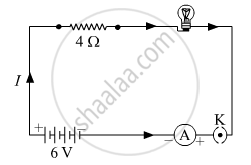# Answer the Following Question. an Electric Lamp of Resistance 20 0 and a Conductor of Resistance 4 0 Are Connected to a 6 V Battery as Shown in the Circuit. Calculate: - Science

Numerical

An electric lamp of resistance 20 Ω and a conductor of resistance 4 0 are connected to a 6 V battery as shown in the circuit. Calculate:(a) the total resistance of the circuit,

(b) the current through the circuit,

(c) the potential difference across the  (i) electric lamp and (ii) conductor, and

(d) power of the lamp.

#### Solution

Resistance of electric lamp = 20 Ω
Resistance of conductor = 4 Ω
Voltage battery = 6 V

(a) Total resistance of circuit = 20 Ω + 4 Ω = 24

(b) Resistance of conductor= 4 Ω
Voltage battery = 6 V
Apply Ohms law
6 V  = "I" × 24 Ω
"I" = (6"V")/(24Ω) = 0.25"A"
Hence, current in the circuit is 0.25A

(c)
(i) Potential difference across the lamp
Vlamp = IR
Vlamp  = 0.25 A × 20 Ω = 5 V
∴ Vlamp = 5 V

(ii) Potential difference across the conductor

"V"_"conductor" = "IR"
"V"_"conductor" = 0.25 "A"xx4 Ω = 1 "V"
"V"_"conductor" = 1"V"

(d) Power of lamp
"I"^(2)"R" = (0.25)^2 xx 20 = 1.25 "W"

Concept: Electric Current
Is there an error in this question or solution?